Test: Controllers & Compensators - 2

# Test: Controllers & Compensators - 2 - Electrical Engineering (EE)

Test Description

## 20 Questions MCQ Test GATE Electrical Engineering (EE) 2024 Mock Test Series - Test: Controllers & Compensators - 2

Test: Controllers & Compensators - 2 for Electrical Engineering (EE) 2023 is part of GATE Electrical Engineering (EE) 2024 Mock Test Series preparation. The Test: Controllers & Compensators - 2 questions and answers have been prepared according to the Electrical Engineering (EE) exam syllabus.The Test: Controllers & Compensators - 2 MCQs are made for Electrical Engineering (EE) 2023 Exam. Find important definitions, questions, notes, meanings, examples, exercises, MCQs and online tests for Test: Controllers & Compensators - 2 below.
Solutions of Test: Controllers & Compensators - 2 questions in English are available as part of our GATE Electrical Engineering (EE) 2024 Mock Test Series for Electrical Engineering (EE) & Test: Controllers & Compensators - 2 solutions in Hindi for GATE Electrical Engineering (EE) 2024 Mock Test Series course. Download more important topics, notes, lectures and mock test series for Electrical Engineering (EE) Exam by signing up for free. Attempt Test: Controllers & Compensators - 2 | 20 questions in 60 minutes | Mock test for Electrical Engineering (EE) preparation | Free important questions MCQ to study GATE Electrical Engineering (EE) 2024 Mock Test Series for Electrical Engineering (EE) Exam | Download free PDF with solutions
 1 Crore+ students have signed up on EduRev. Have you?
Test: Controllers & Compensators - 2 - Question 1

### Assertion (A) : The phase lag network provides an attenuation of 20 log a (a < 1) at high frequencies having transfer function equal to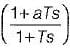Reason (R): The lag network allows to pass high frequencies and low frequencies are attenuated.

Detailed Solution for Test: Controllers & Compensators - 2 - Question 1

Here, reason (R) is false because the lag network allows to pass low frequencies and high frequencies are attenuated.

Test: Controllers & Compensators - 2 - Question 2

### Assertion (A): Introduction of phase lag network in forward path increases the phase shift. Reason (R): A phase lag network has pole nearer to the imaginary axis as compared to zero.

Detailed Solution for Test: Controllers & Compensators - 2 - Question 2

The transfer function of a phase-lag network is: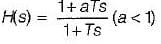The pole-zero plot is shown below: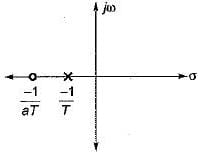Here, pole is nearer to the imaginary axis and origin compared to zero.
Hence, reason (R) is true.
Introduction of phase lag network in forward path reduces the phase shift. Hence, assertion (A) is a false statement.

Test: Controllers & Compensators - 2 - Question 3

### Match List-I (Type of controllers) with List-ll (Alternate names) and select the correct answer using the codes given below the lists: List-I A. Two-position controller B. Proportional controller C. Integral controller D. Derivative controller List-II 1. Rate controller 2. Reset controller 3. An amplifier with adjustable gain 4. ON-OFF controller Codes:     A B C D (a) 4 2 3 1 (b) 4 3 2 1 (c) 3 4 2 1 (d) 3 4 1 2

Test: Controllers & Compensators - 2 - Question 4

The open loop transfer function of a three action controller (PID controller) is given by

Detailed Solution for Test: Controllers & Compensators - 2 - Question 4

A PID controller is shown below.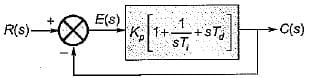Here,
G(s) = O.L.T.F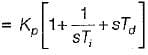Test: Controllers & Compensators - 2 - Question 5

The action of a PD controller is to

Detailed Solution for Test: Controllers & Compensators - 2 - Question 5

Due to PD controller, a zero is added to the forward path which results in a lead compensator. Therefore, steady state error remais the same whiie rise time of the system is reduced (i.e. stability is increased).

Test: Controllers & Compensators - 2 - Question 6

For the derivative feedback control shown in figure below, the damping ratio is equal to 0.7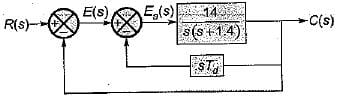The value of derivative gain constant is

Detailed Solution for Test: Controllers & Compensators - 2 - Question 6

For the given system, characteristic equation is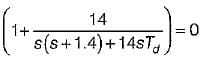or,
s2 + 1.4s + 14s Td + 14 = 0
or,
s2 + (1.4 + 14Td) s + 14 = 0
Comparing with s2 + 2 ξωn + ωn2 =0, we have:
ωn = √14 rad/s and 2ξωn = (1.4 + 14Td)
or, 2 x 0.7 x √14 = 1.4 + 14 Td (∴ ξ = 0.7, given)
or,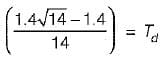or,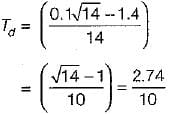= 0.274

Test: Controllers & Compensators - 2 - Question 7

The block diagram of an integral control is shown in figure below.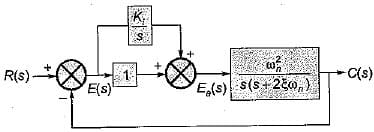The steady state error in the above system for a parabolic input is

Detailed Solution for Test: Controllers & Compensators - 2 - Question 7

For the given system, forward path transfer function is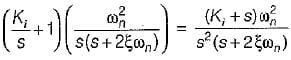For parabolic input, steady state error is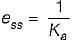where,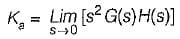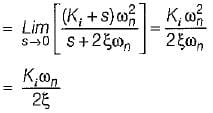∴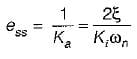Test: Controllers & Compensators - 2 - Question 8

A system employing proportional plus error rate control is shown in figure below.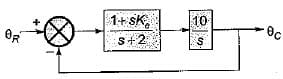The value of error rate control (Ke) and 2% settling time for a damping ratio of 0.5 are respectively

Detailed Solution for Test: Controllers & Compensators - 2 - Question 8

The forward path gain of the given system is: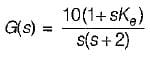∴ Characteristic equation is
1 + G(s)H(s) = 0
or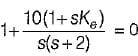or, s2 + (2 + 10 Ke)s +10 = 0
Here,
and 2ξω= (2 + 10Ke)
Given, ξ = 0.5
So, 2 x 0.5 x √10 = (2 + 10Ke)
or,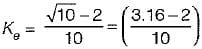= 1.16/10 = 0.116
Also, 2% settling time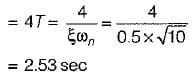Test: Controllers & Compensators - 2 - Question 9

A feedback control system employing output- rate damping is shown in figure below.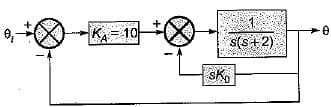What is the steady state error (in radian) for the above system resulting from an unit ramp input if the damping ratio is 0.6?

Detailed Solution for Test: Controllers & Compensators - 2 - Question 9

The characteristic equation of the given system is
1+G(s) H(s) = 0
Here,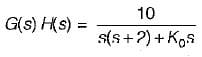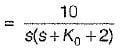∴ Characteristic equation is
10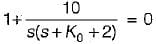or, s2 + (K0 + 2)s +10 = 0
Comparing with s2 + 2ξωns + ωn2 = 0 we have
and 2ξωn = K0 + 2
Given, ξ = 0.6
∴ 2 x 0.6 x √10 = K0 + 2
or, K0 = 1.792
For a unit ramp input, ess = 1/Kv
where,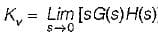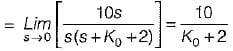∴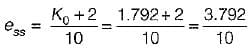Test: Controllers & Compensators - 2 - Question 10

In the control system shown below, the controller which can give infinite value of steady-stae error to a ramp input is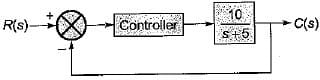Detailed Solution for Test: Controllers & Compensators - 2 - Question 10

Case-1: For integral type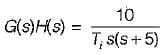∴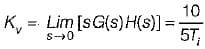∴ ess = Ti/2
Case-2: For derivative type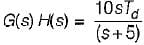G(s)H(s) = (10sTd)/(s+5)
∴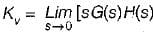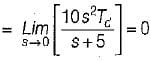∴ ess = 1/Kv =
Case-3: For proportional type
G(s)H(s) = 10Kp/(s+5)
∴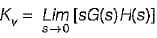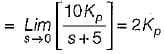∴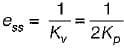Case-4: For proportional plus derivative type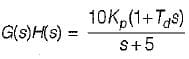∴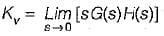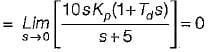∴ ess = 1/Kv = ∞

Test: Controllers & Compensators - 2 - Question 11

The transfer function of the OP-AMP circuit shown below is given by: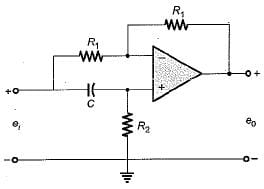Detailed Solution for Test: Controllers & Compensators - 2 - Question 11

Redrawing the given circuit in s-domain, we have: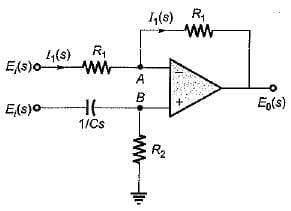Now,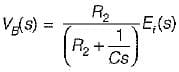From virtual ground concept,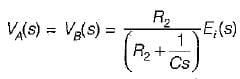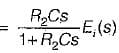Now,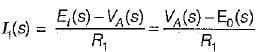or,
2VA(s) = Ei(s) + E0(s)
E0(s) = 2VA(s) - Ei(s)
or,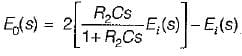or,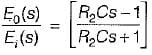Test: Controllers & Compensators - 2 - Question 12

The transfer function of a phase lead compensator is given by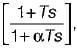where α < 1 and T> 0.
Which of the following is not a correct value of maximum phase shift provided by such a compensator?

Test: Controllers & Compensators - 2 - Question 13

Assertion (A): Stepping motors are compatible with modern digital systems.
Reason (R): The nature of command is in the forms of pulses.

Detailed Solution for Test: Controllers & Compensators - 2 - Question 13

Since the nature of command is in the form of pulses, therefore stepping motors are compatible with modern digital systems.

Test: Controllers & Compensators - 2 - Question 14

Consider the control system shown in figure below employing a proportional compensator.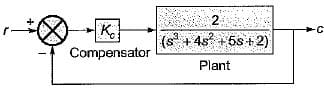If the steady-state error has to be less than two percent for a unit step input, then the value of Kc would be

Detailed Solution for Test: Controllers & Compensators - 2 - Question 14

For given system,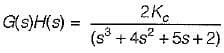With proportional controller,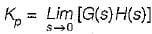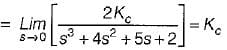∴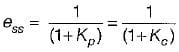(for a unit step input)
Given, ess = less than 2% of input
i.e. ess < 0.02
Now, characteristic equation is:
1 + G(s)H(s) = 0
or, s3 + 4s2 + 5s + 2 + 2Kc = 0
Routh’s array is: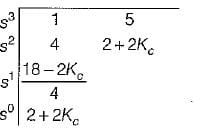For stability of given system,
2 + 2Kc > 0 or Kc > -1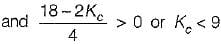or Kc < 9
Hence, -1 < Kc < 9 (for stability) ....... (1)
Now,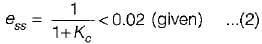From equations (1) and (2), we conclude that the minimum value of steady state error = 0.1 when Kc = 9.
Also, for all value of Kc between -1 and 9, value of ess will be more than 0.02 i.e. more than 2% of input.
Therefore, there is no such value of Kfor given system i.e. can not be determined.

Test: Controllers & Compensators - 2 - Question 15

Match List-I (Controllers) with List-II (Transfer functions) and select the correct answer using the codes given below the lists: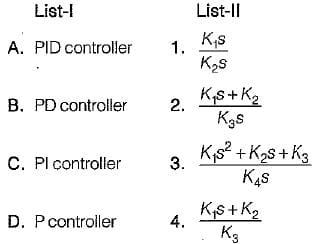Codes:
A B C D
(a) 2 3 4  1
(b) 3 4 1  2
(c) 3 4  2 1
(d) 3 1 4  2

Test: Controllers & Compensators - 2 - Question 16

The transfer function of a system is given as: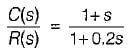It represents a

Detailed Solution for Test: Controllers & Compensators - 2 - Question 16

Given,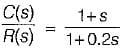The pole-zero plot is shown below.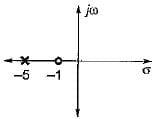Since zero is near to origin, therefore it represents a lead network.

Test: Controllers & Compensators - 2 - Question 17

Integral feedback control

Test: Controllers & Compensators - 2 - Question 18

The industrial controller having the best steady state accuracy is

Test: Controllers & Compensators - 2 - Question 19

The transfer function of a phase lead controller is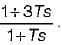The maximum value of phase shift provided by this controller is

Detailed Solution for Test: Controllers & Compensators - 2 - Question 19

Given,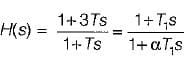Here, T1 = 3T and αT1 = T
∴ α = T/T= T/3T = 1/3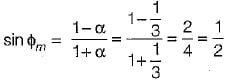or,  ϕm = 30° = maximum phase shift

Test: Controllers & Compensators - 2 - Question 20

With regard to the filtering property, the lead compensator and the lag compensators are respectively

## GATE Electrical Engineering (EE) 2024 Mock Test Series

23 docs|285 tests
Information about Test: Controllers & Compensators - 2 Page
In this test you can find the Exam questions for Test: Controllers & Compensators - 2 solved & explained in the simplest way possible. Besides giving Questions and answers for Test: Controllers & Compensators - 2, EduRev gives you an ample number of Online tests for practice

## GATE Electrical Engineering (EE) 2024 Mock Test Series

23 docs|285 tests